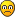## Recommended Posts

So, I'm trying to re-work WX-78's rate of wetness damage, so that it would increase (be faster) the more wetness he has. So, this is what I have:

```--Declares a variable that is the current moisture of the player character
local wetness_percent = inst.components.moisture:GetMoisture()

--Determines how long until the next spark happens
inst.spark_time_offset = (3 *(1 -wetness_percent)) + 1```

The code above would mean that at just above 0 wetness, the rate would be 4 seconds, while being 1 second at full wetness. That is if 100% = 1 in terms of numbers.

However, when testing it, just above 1 wetness, the rate starts to become extremely fast. Why is that? Any way I can fix this and make this work correctly?

I've been trying to get this working for like an hour now and no amount of change fixes it. It's either too slow at times or way too fast##### Share on other sites

I've found the issue:

`inst.components.moisture:GetMoisture()`

^ that part of code takes the amount, not percentage, so its value is 100 and not 1 in numbers. What I needed to do is set the code to (3*(100 -wetness_percent)) and then divide all of that by 100. Of course, adding the 1 or whatever other sum I want at the end, which would indicate the smallest interval amount that is possible (so that it isn't rappid at 100 wetness).